Support the Monkey! Tell All your Friends and TeachersHome MonkeyNotes Printable Notes Digital Library Study Guides Study Smart Parents Tips College Planning Test Prep Fun Zone Help / FAQ How to Cite New Title Request

 8.3 Roots of Complex Numbers Given a complex number z = r (cos q + i sin q) . All the roots of 'z' are given by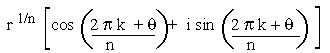where k = 0, 1, 2, 3, ...., (n-1) i) If k = 0 then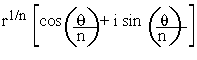which is the principal nth root of z ii) Also, for q = 0 and r = 1 then z = 1 and nth root of unity are given by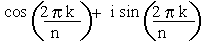,k = 0, 1, 2, 3, ...., (n - 1) Example 1 Find three cube roots of unity. Solution n = 3 (1)1/3 =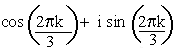Thus z3 = 1 and k = 0, 1, 2 when k = 0 z1 = cos (0) + i sin (0) z1 = 1 when k = 1, z1 =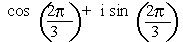= cos (1200) + sin (1200)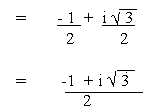when k = 2 , z3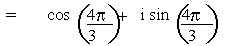= cos (2400) + i sin (2400)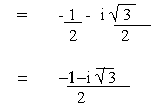Hence the three cube roots of unity are 1,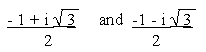Note that these roots are also denoted by 1, w and w2 respectively Also, not that they are in geometric progression and we have l + w + w2 = 0, w3 = 1 etc. Example 2 Prove that the nth roots of unity form a G.P and each can be shown as power of the other. Solution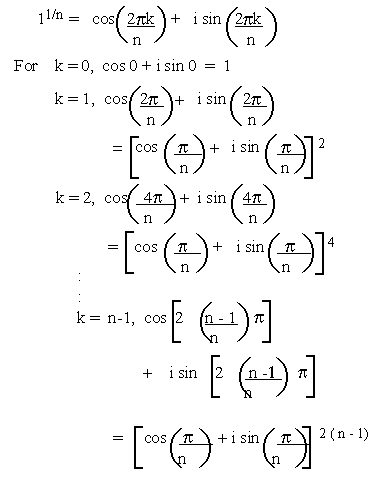Let these roots be denoted by 1, w, w2......, wn -1 forms a G. P. with common ratio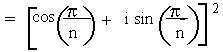Index Chapter 9
 Search: All Products Books Popular Music Classical Music Video DVD Toys & Games Electronics Software Tools & Hardware Outdoor Living Kitchen & Housewares Camera & Photo Cell Phones Keywords: